# Linear Inequalities NCERT Solutions : Class 11 Maths

## NCERT Solutions for Class 11 Maths : Linear InequalitiesFilter
Filters :
Classes
•
•
•
•
•
•
•
Chapters
•
•
•
•
•
•
•
•
•
•
• 6 More
Exercises
•
•
•
•
•

Doubtnut one of the best online education platform provides free NCERT Solutions of Maths for Class 11 Linear Inequalities which are solved by our maths experts as per the NCERT (CBSE) guidelines. We provide the solutions as video solutions in which concepts are also explained along with answer which will help students while learning , preparing for exams and in doing homework. These Solutions will help to revise complete syllabus and score more marks in examinations.

Get here free, accurate and comprehensive NCERT Solutions for Class 11 Maths Linear Inequalities which have been reviewed by our maths counsellors as per the latest edition following up the CBSE guidelines. We provide video solutions in which solutions to all the questions of NCERT Class 11 Maths textbook are explained in step by step and detailed way.

## The Topics of the Chapter Linear Inequalities are :

SOLVING LINEAR INEQUATION IN ONE VARIABLE

GRAPHICAL SOLUTION OF LINEAR INEQUATION IN ONE VARIABLE

SOME APPLICATIONS OF LINEAR INEQUATION IN ONE VARIABLE

SOLUTION OF SIMULTANEOUS LINEAR INEQUATION IN TWO VARIABLE

SOLUTION OF AN INEQUATION

INTRODUCTION

It contains these exercises along with solved examples. All the exercises are solved in the video. Select the exercise to view the solutions exercisewise of the Chapter Linear Inequalities:

## NCERT Solutions Class 11 Maths Chapter Linear Inequalities Exercises:

We have covered all the exercises and also Solved examples in the videos. Along with the practise exercise Students should also practise solved examples to clear the concepts of the Linear Inequalities. If incase you have any doubt you can watch the solutions for the given questions.Watch the solutions for the given questions in which it is also explained in the video steps to solve the questions along with answers.

We here in this chapter will be dealing with inequalities in the linear function. A linear inequality contains one of the symbols of inequality. It has the data, which is not equal in graph form.

< is less than

> is greater than or equal to

→≤ is less than or equal to

→≥ is greater than or equal to

→≠ is not equal to

→= is equal to

It is an interesting chapter as here we will get to know what is the actual difference between linear inequality and linear equation which is linear equation x+3 = 2 and linear inequality which has the less-than sign instead of n equals sign which makes all the difference. The basic steps that are to be taught in this chapter as to how to calculate the linear inequalities, which has three steps:

1. Rearrange the equation so “y” is on the left and everything else on the right.

2. Plot the “y” = line (make it a solid line for y≤ or y ≥, and a dashed line for y< or y>)

3. The shade above the line for a “greater than” (y> or y≥) or below the line for a “less than” (y< or y ≤).

We will deal with everything related to the chapter here which is included in the syllabus issued by the council. We make sure to go across every topic included and not miss any of the concepts. Moreover, you can visit Doubtnut anytime for more comprehensive solutions and video tutorials on the same.

NCERT book provides the best and most authentic content that you will find in the market for academics and even from the competition point of view as most of the questions formed and framed in the question paper has its reference more or less from the NCERT text. Therefore, it is beneficial to read NCERT books for class 11  to do well in exams. NCERT provides a question bank after the completion of each topic so that you can practice more efficiently. They have a number of concepts explained in the most appropriate amount in a single paragraph as needed by you and they avoided unnecessary explanations anywhere in the content. Thus, keeping the book look less scary for you to read it willingly. They have coloured printing of graphs, diagrams, and tables for the best explanation as well as it keeps you interested in the topic and does not bring that boring black theme. The content provided is put together by best authors, who have years of experience and good command on the subject. So, you need not to worry as each and every topic is covered in the book.

For more, you can visit us through our website Doubtnut to discover fun and interesting way of learning. We provide video solutions for your in-depth understanding of the mathematical concept. At Doubtnut, we make sure to make use of easy and simple language that everyone can understand. Also, appropriate use of graphics and diagrams has been done to inculcate the readers and learners with the best experience. You will find the website entertaining and knowledgeable at the same time as we try to provide the best learning material in an interactive manner along with precision.

## Topics & Subtopics of NCERT Class 11 Maths Chapter 6 – Linear Inequalities

### Linear Inequalities

OVERVIEW:

In this chapter, we will study linear inequation in one and two variables. The knowledge of linear inequation is very helpful in solving problems in science, mathematics and in linear programming, etc.

The solution of a linear inequality in two variables like Ax+By >C is an ordered pair (x, y) that produces a true statement when the values of x and y are substituted into the inequality. The graph of an inequality in two variables is the set of points that represents all solutions to inequality.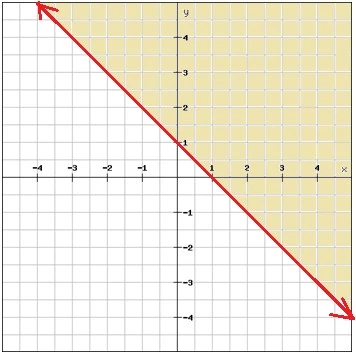### Solution to Inequalities

We can find the solution to inequalities by just checking the endpoints, in the question given to us. Picking a value greater than, such as 2, to check in the inequality. Dividing both the sides by three (3) to isolate the variable. Check the solution by first checking the endpoint 4, and then checking another solution for the inequality.

Solving an inequation – it is the process of obtaining all possible solutions of an inequation solution set- the set of all possible solutions of an inequation is known as its solution set.

EXAMPLE:

1. Simplify:

x+2-2>12-2

x>10 (solution)

WAYS TO SOLVE THEM:

1. Add/subtract a number from both the sides.

2. Multiply or divide both the sides of the inequality sign by a positive number.

3. Simplify a side.

Example:

We can simplify 7+3 without even changing the inequality:

3x<10

But these things do not change the direction of the inequality (‘’<’’ becomes ‘>’ for example).

Multiply or divide both the sides by a negative number

Swapping/switching left and right-hand sides.

Example:

2y+7<12

And when we switch the right and left and side, we must also change the direction of the inequality

12>2y+7.

## Representation of Solution Linear Inequality in One Variable on A Number Line

We can also graph inequalities in one variable on the number line. The following graph represents the inequality x≤3. The dark line represents all the numbers that satisfy x≤3. If we pick any number on the dark line and put it in for x, the inequality will be true.

### Graphical Representation of Solution of Linear Inequalities in Two Variables

If there are two variables in a linear inequality in two variables:

EXAMPLE:

2x+3y>50

The solution of a linear inequality in two variables like ax+by>c is an ordered pair (x, y) that gives a true statement when we put the values of x and y into the inequality. We will solve the linear inequality, with the same method of solving the linear equation:

EXAMPLE:

x+9y<27

Let x=0

0+9y<27

9y<27

y<3

Let y=0

x+9(0) <27

x<27

So the solution of the given inequality are (0, 3) and (27, 0).

This shows that if x=0 then y could be less than 3 that is 0, 1, 2. We will not include 3 here as it is less than three only not less than or equal to three. The solution set for this are:

(0, 0),(0, 1),(0, 2)

All the coordinates of these ordered pairs will come in the solution part.

The same way we can find the solution set by assuming the value of x as 1, 2, 3 and so on.

### Graphical Solution of Linear Inequalities in Two Variables

The graph of inequality is the visual representation of the solution of the inequality.

The solution of the linear inequality with two variables is represented by the half-plane on the Cartesian or coordinate plane.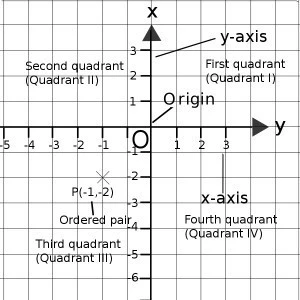### What is Half Plane

1. A vertical line divides the plane between the left half-plane and right half-plane.

2. A non-vertical line divides the plane in upper half-plane and lower half-plane.

3. The line of equation, which divides the plane, is called the boundary line.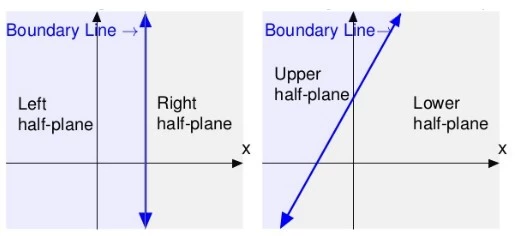Two Important Results :

A.   a,b ÏµR  and b ≠0, then

1- ab >0 or a/b >0 this implies that a and b are of the same sign

2- ab <0 or a/b <0 this implies that a and b are of opposite sign

B. if a is any positive real number that is a>0, then

1- Ë©xË©

Ë©xË© ≤ a, this implies that -a ≤a

2- Ë©xË© > a this implies that x<-a or x>a

Ë©xË© a, this implies that x -a or xa

Case 1. When -

Ë©x+1Ë© + Ë©xË©>3 this implies x1-x >3 this implies that x<-2

Case 2. -1x<0

Ë©x+1Ë© + Ë©xË©>3 this implies that x+1-1>3 this implies that 1>3

Case 3. When 0x<

Ë©x+1Ë© +Ë©xË© >3 this implies that x+1+x>3 this implies that x>1

Combining the results of cases 1, 2, 3 we get

x belongs to (-∞,-2)U(1,∞)

### Graphs of Linear Inequalities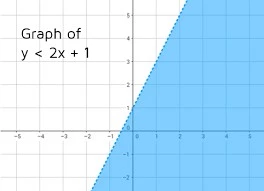The linear inequation in the graph is y<2x+1.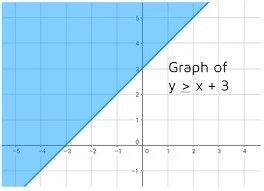The linear inequation in the graph is y≥x+3.

## Benefits of Doubtnut

1. Connect to us through our website to get the best and easiest methods to solve the exercise questions of the NCERT book.
2. We even provide video lectures conducted by the well-versed experts with good experience and good training in imparting their knowledge in the way you can understand. At Doubtnut, we explain everything with a good example of the easy and practical understanding of the concept.
3. Moreover, you can always post queries to get solutions in seconds, all you have to do is click a photo of doubt question from the maths book and post it on our website or app.
4. You will also find the pdf format of the NCERT solutions provided at the website, which is easily accessible to everyone.
5. The language used while explaining concepts is kept simple and lucid for you to understand better.

Share this on :-